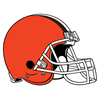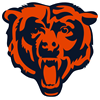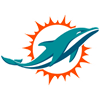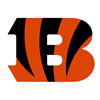PointsBet 2020 Super Bowl Odds
+500
5 to 1Chiefs
13.2% implied probability

+600
6 to 1Ravens
11.3% implied probability

+900
9 to 149ers
7.9% implied probability

+1000
10 to 1Buccaneers
7.2% implied probability

+1100
11 to 1Saints
6.6% implied probability

+1700
17 to 1Cowboys
4.4% implied probability

+2000
20 to 1Seahawks
3.8% implied probability

+2000
20 to 1Eagles
3.8% implied probability

+2000
20 to 1Patriots
3.8% implied probability

+2200
22 to 1Colts
3.4% implied probability

+2500
25 to 1Vikings
3.0% implied probability

+2500
25 to 1Steelers
3.0% implied probability

+2500
25 to 1Bills
3.0% implied probability

+3000
30 to 1Titans
2.6% implied probability

No changes have been recorded yet.
+3000
30 to 1Packers
2.6% implied probability

+3300
33 to 1Browns
2.3% implied probability

+3300
33 to 1Rams
2.3% implied probability

+4500
45 to 1Falcons
1.7% implied probability

+5000
50 to 1Bears
1.6% implied probability

+5000
50 to 1Broncos
1.6% implied probability

+5000
50 to 1Chargers
1.6% implied probability

+5500
55 to 1Cardinals
1.4% implied probability

+5500
55 to 1Raiders
1.4% implied probability

+6600
66 to 1Texans
1.2% implied probability

+8000
80 to 1Jets
1.0% implied probability

+8500
85 to 1Dolphins
0.9% implied probability

+10000
100 to 1Lions
0.8% implied probability

+12500
125 to 1Bengals
0.6% implied probability

No changes have been recorded yet.
+12500
125 to 1Giants
0.6% implied probability

+15000
150 to 1Panthers
0.5% implied probability

+15000
150 to 1Redskins
0.5% implied probability

No changes have been recorded yet.
+20000
200 to 1Jaguars
0.4% implied probability